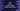# Create a random integer, double or a boolean in dart using Random class# Introduction :

Like most other programming languages, dart has one built-in random number generator class. It is defined in the dart:math library package. You can create one random integer, double or boolean using this random number generator class. In this post, I will show you how to do that with different examples. Let’s have a look :

## Random class :

Random class is used to create a random value in dart. As I have mentioned before, it is defined in dart:math library. We can create one random boolean, integer or double using this class. It has two different constructors. The default one is not secure or we can’t use it for cryptographic purpose. For cryptographic usage, it provides one separate constructor.

## Constructors of Random class :

Following are the two constructors of the Random class :

``Random([int seed])``

This constructor creates one random number generator. The parameter seed is an optional value. It is used to initialize the internal state of the generator.

``Random.secure()``

This is the second constructor. It can create one cryptographically secure random number generator. It throws one UnsupportedError if it fails.

## Methods :

Basically, we have three methods for the random value generation. We can create one random boolean, integer or double.

### 1. nextBool() :

It is used to create a random boolean value.

### 2. nextDouble() :

It is used to create a random floating-point value. This value is uniformly distributed in the range of 0.0(inclusive) to 1.0(exclusive).

### 3. nextInt(int max) :

Returns one random non-negative integer. This is distributed in the range from 0(inclusive) to max(exclusive). The value of max can be in the range of 1(inclusive) to 1<<32(exclusive).

## Example Program :

``````import 'dart:math';

void main() {
print("Using Random()");
Random r1 = new Random();
print(r1.nextInt(100));
print(r1.nextBool());
print(r1.nextDouble());

print("\nUsing Random.secure() :");
Random r2 = new Random.secure();
print(r2.nextInt(100));
print(r2.nextBool());
print(r2.nextDouble());
}``````

It will print output like below :